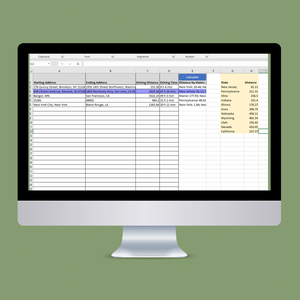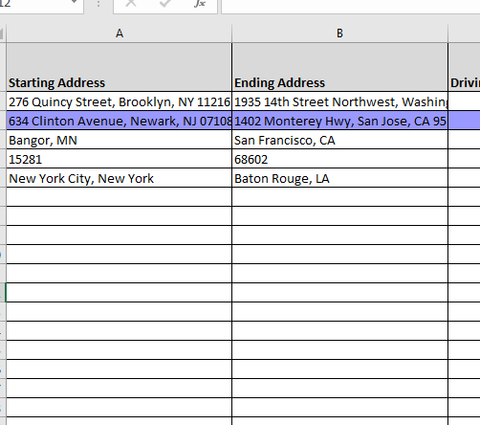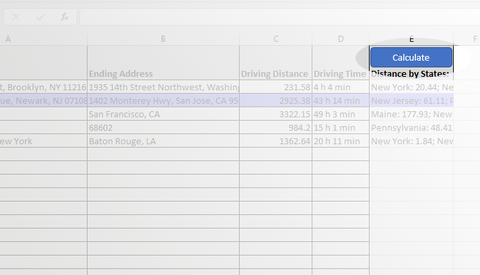# IFTA Miles Excel Distance Calculator

Regular price
\$67.00
Sale price
\$67.00

## What is the IFTA Miles Excel Distance Calculator?

The IFTA Miles Excel Distance Calculator is an Excel-based tool that allows the user to do the following

• Generate Total Driving Miles and Total Driving time between 2 addresses or zip codes.
• Generate Miles driven for each state during the trip.

### Why is this important?

Many transportation companies need to calculate the miles driven during the trip for each state for regulatory purposes such as IFTA or State Taxes.

### How do I use the "IFTA Miles Excel Distance Calculator"?

To use this tool is extremely easy.

#### Step 1 - Paste the To and From Address as you would usually do.#### Step 2 - Just hit Calculate. The tool will do the following 2 thingsIt will calculate Total Driving Distance and Total Driving Time between each of the 2 points.(as indicated in purple_

It will calculate the miles traveled by State for each trip (as indicated in yellow)Q and A
Q: Will this work outside of the United States?
A: While the distance calculation part will work outside of United states, the state-by-state distance only works within US. If you are just looking for a regular distance calculator click here to go to our Distance Calculator Page

Q: Can I calculate the distance between exact addresses, not just Zip Codes?
A: Yes, the calculator can handle partial addresses as well as complete addresses. (Cities, States, Countries, etc.)

Q: Does this calculator Calculate Driven Distance, or Straight Line (as the crow flies) distance.
A: All the calculations are done using Google Maps API and the distance generated is Driven Miles/Distance. The results are up to date and generated as you would by searching in Google Maps

Q: Will this work on a Mac as well as a Windows Machine?
A: Yes, the calculator will work on recent versions of Excel for Mac as well as Windows.

Q: Are there any limitations?
A: Yes, driving distance is generated using Google Maps API which limits the free results to 40,000 calculations per month. After that, Google charges 5 USD per 1000 calculations. Get more information regarding the Google Maps API here.